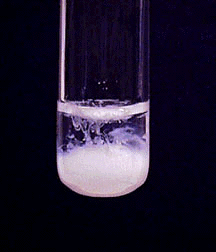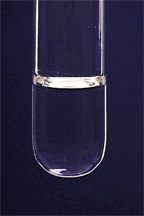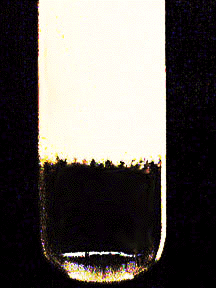# Characteristic Reactions of Lead Ions (Pb²⁺)

$$\newcommand{\vecs}{\overset { \rightharpoonup} {\mathbf{#1}} }$$ $$\newcommand{\vecd}{\overset{-\!-\!\rightharpoonup}{\vphantom{a}\smash {#1}}}$$$$\newcommand{\id}{\mathrm{id}}$$ $$\newcommand{\Span}{\mathrm{span}}$$ $$\newcommand{\kernel}{\mathrm{null}\,}$$ $$\newcommand{\range}{\mathrm{range}\,}$$ $$\newcommand{\RealPart}{\mathrm{Re}}$$ $$\newcommand{\ImaginaryPart}{\mathrm{Im}}$$ $$\newcommand{\Argument}{\mathrm{Arg}}$$ $$\newcommand{\norm}{\| #1 \|}$$ $$\newcommand{\inner}{\langle #1, #2 \rangle}$$ $$\newcommand{\Span}{\mathrm{span}}$$ $$\newcommand{\id}{\mathrm{id}}$$ $$\newcommand{\Span}{\mathrm{span}}$$ $$\newcommand{\kernel}{\mathrm{null}\,}$$ $$\newcommand{\range}{\mathrm{range}\,}$$ $$\newcommand{\RealPart}{\mathrm{Re}}$$ $$\newcommand{\ImaginaryPart}{\mathrm{Im}}$$ $$\newcommand{\Argument}{\mathrm{Arg}}$$ $$\newcommand{\norm}{\| #1 \|}$$ $$\newcommand{\inner}{\langle #1, #2 \rangle}$$ $$\newcommand{\Span}{\mathrm{span}}$$

• Most common oxidation states: +2, +4
• M.P. 328º
• B.P. 1750º
• Density 11.35 g/cm3
• Characteristics: Lead is a soft metal having little tensile strength, and it is the densest of the common metals excepting gold and mercury. It has a metallic luster when freshly cut but quickly acquires a dull color when exposed to moist air.
• Characteristic reactions of $$\ce{Pb^{2+}}$$: The +2 oxidation state is the more stable state.

## Chloride Ion

Soluble chlorides, such as hydrochloric acid, precipitate white lead chloride from $$\ce{Pb^{2+}}$$ solutions, when the solutions are not too dilute:

$\ce{Pb^{2+}(aq) + 2Cl^{-}(aq)<=> PbCl2(s) }$Lead chloride is a slightly soluble salt, with a solubility of 10 g/L at 20º. The solubility of $$\ce{PbCl2}$$ increases very rapidly as the temperature rises. At 100º it has a solubility of 33.5 g/L. However, $$\ce{PbCl2}$$ precipitates very slowly, particularly when other ions that form insoluble chlorides are not present. The precipitation can be speeded up by vigorously rubbing the inside of the test tube with a stirring rod. Even then the precipitate may not form until 3 to 5 minutes after mixing the solutions. $$\ce{PbCl2}$$ dissolves in excess chloride ion as a result of the formation of the tetrachloroplumbate(II) complex ion:

$\ce{PbCl2(s) + 2Cl^{-}(aq)<=> [PbCl4]^{2-}(aq) }$## Sulfate Ion

Soluble sulfates, including dilute sulfuric acid, precipitate white lead sulfate, which is much less soluble than lead chloride:

$\ce{Pb^{2+}(aq) + SO4^{2-}(aq)<=> PbSO4(s) }$$$\ce{PbSO4}$$ dissolves in concentrated solutions of hydroxide or acetate ions.

$\ce{PbSO4(s) + 4OH^{-}(aq)<=> [Pb(OH)4]^{2-}(aq) + SO4^{2-}(aq) }$

$\ce{PbSO4(s) + 2CH3CO2^{-}(aq)<=> Pb(CH3CO2)2(aq) + SO4^{2-}(aq) }$The lead acetate, though only slightly dissociated, is soluble.

## Aqueous Ammonia

Lead(II) ion reacts with aqueous ammonia to precipitate a white basic salt, $$\ce{Pb2O(NO3)2}$$, rather than the expected lead(II) hydroxide:

$\ce{Pb^{2+}(aq) + 2NH3(aq) + 3H2O(l) + 2NO3^{-}(aq)<=> Pb2O(NO3)2(s) + H2O(l) + 2NH4^{+}(aq) }$The basic salt is insoluble in excess ammonia.

## Sodium Hydroxide

Sodium hydroxide precipitates lead(II) hydroxide, which dissolve with excess hydroxide:

$\ce{Pb^{2+}(aq) + 2OH^{-}(aq)<=> Pb(OH)2(s) }$$\ce{Pb(OH)2(s) + 2OH^{-}(aq)<=> [Pb(OH)4]^{2-}(aq) }$Characteristic Reactions of Lead Ions (Pb²⁺) is shared under a not declared license and was authored, remixed, and/or curated by James P. Birk.